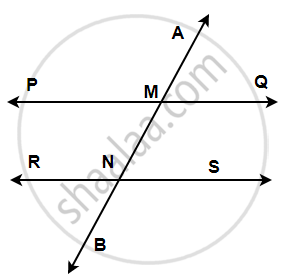# Properties of Parallel Lines - Corresponding Angle Theorem

#### Topics

• Theorem: If a transversal intersects two parallel lines, then each pair of corresponding angles is equal.

## Theorem

#### If a transversal intersects two parallel lines, then each pair of corresponding angles is equal.

This is also referred to as the corresponding angles axiom.Given: Two Parallel lines PQ and RS.

Let AB be the transversal intersecting PQ at M and RS and N.

To Prove: Each pair of corresponding angles are equal.

i.e., ∠ AMP ≅ ∠ MNR, ∠ PMN ≅ ∠ RNB,

and ∠ AMQ ≅ ∠ MNS, ∠ QMN ≅ ∠ SNB.

Proof:

First, we will prove ∠ AMP ≅ ∠ MNR.

For lines PQ and RS  with transversal AB,

∠QMN = ∠MNR        ......(Alternate Interior angles)(1)

For lines PQ and AB,

∠AMP = ∠QMN       .......(Vertically opposite angles)(2)

From (1) and (2),

∠AMP = ∠MNR

Similarly, we can prove that

∠ PMN ≅ ∠ RNB,

∠ AMQ ≅ ∠ MNS,

∠ QMN ≅ ∠ SNB.

Hence, Each pair of corresponding angles are equal.

If you would like to contribute notes or other learning material, please submit them using the button below.

### Shaalaa.com

Axiom: If a Transversal Intersects Two Parallel Lines, Then Each Pair of Corresponding Angles is Equal. [00:03:38]
S
0%